Wednesday 21st October 2020CBSE Guess > Papers > Important Questions > Class XII > 2009 > Maths > Mathematics By Anil Kumar Tondak

CBSE CLASS XII

Vector Algebra

Q. 1. Find a vector in the direction of vector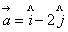that has magnitude 7 units.

Q. 2. Show that the points A, B and C  with position vectors,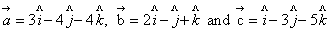respectively, form the vertices of a right angled triangle.

Q. 3. Find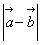,  if two vectors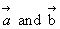are such that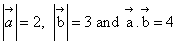.

Q. 4. Find the area of the parallelogram whose adjacent sides are determined by the vectors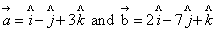Q. 5. If a unit vector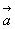makes angles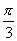with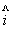,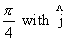and acute angle θ with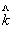, then find θ and hence
the components of.

Q. 6. Let,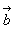and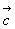be three vectors such that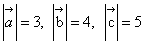and each one of them being perpendicular to the sum of other two, Find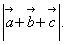Q. 7. Find the value of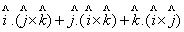Q. 8. The scalar product of the vector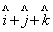with a unit vector along the sum of vectors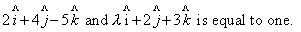Find the value of l.

Q. 9. If the sum of two unit vectors is a unit vector, Prove that the magnitude of their difference is.
Q. 10. Ifare position vectors of points A and B respectively, then find the position vector of points of trisection of AB.

Q. 11. Prove that the line segment joining the mid-points of two sides of a triangle is parallel to the third side and equal to half of it.

Q. 12. ABCD is a parallelogram. If the coordinates of A, B, C are (-2, -1), (3, 0) and (1, -2) respectively, Find the co-ordinate of D.

Q. 13. Show that the points A, B, C with position vectors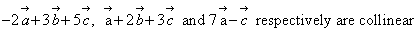Q. 14. If a vector makes a, b,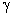with OX, OY and OZ  respectively, prove that sin2a+sin2b+sin2γ =2.
Q. 15. If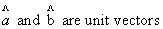inclined at an angle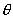, then prove that sin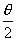=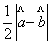.

Q. 16. If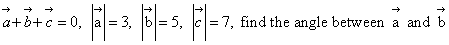.
Q. 17. If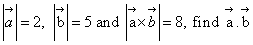.

Prepared By Anil Kumar Tondak
M.Sc.(Maths), M.Ed., M.A.(Eco),M.B.A.(Mkt)
Mob No. 9811363962

 Maths